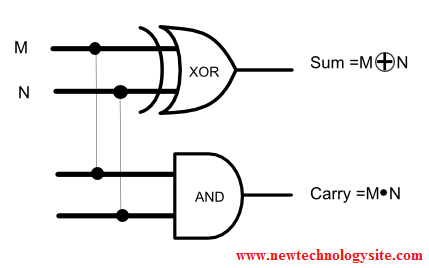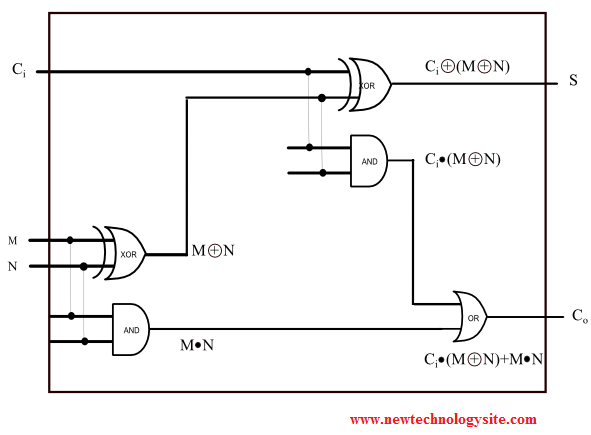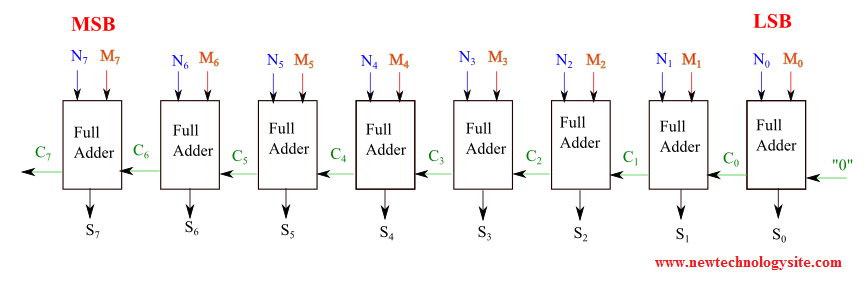# Full Binary Adder in a Computer

## 1- Adding Two One-bit Numbers M and N - Half Adder

We first review adding two decimal (base 10) numbers before considering adding binary (base 2) numbers.
Numbers may be written at any base. Base 10 (decimal system) using the digits: 0, 1, 2, 3, 4, 5, 6, 7, 8 and 9, is the one we use in our everyday life. To add two decimal numbers such as 19 and 26,

 1 9 + 2 6

We start from the right digits (units) 9 and 6. Adding 9 and 6, gives 15 , but because 15 is greater than 10 (the base), we keep the 5 in the same column as 9 and 6 but the "1" is carried and added to the next digits (1 and 2) to the left of the first digits. We then continue with the next digits moving to the left till the last digits.

 1 1 9 + 2 6 _ ___ ___ 5

In computers, the binary system (or base 2) is used. Two digits (0 and 1) are needed to write numbers.
We consider adding two binary (base 2) numbers M and N with one bit each.
1) 1 + 1 = ?
We start from the right: 1 + 1 = 1 0.

 1 1 + 1 ___ ___ ___ 1 0

Explanation: 1 + 1 = 2 in decimal and 2 written in binary is 10 (2 = 1*21 + 0*20)
We put the "0" under the digits on the right and carry the "1" as shown above.

In the above, "0" is called the bit sum and "1" is called the carry.

More additions of one-bit numbers are shown below.
1 + 0 = 1; bit sum is 1 and the carry is 0.
0 + 0 = 0; bit sum is 0 and carry is 0.
0 + 1 = 1; bit sum is 1 and carry is 0.
The above binary additions are put together in the table below.

 Input Output M N Carry Sum 0 0 0 0 0 1 0 1 1 0 0 1 1 1 1 0

It is easy to note that the "Sum" corresponds to the output of an exclusive OR (XOR) logic gate and the "Carry" corresponds to the output of an AND logic gate. Hence the following simplified circuit could be used to add two bits and also generate the carry.Figure 1. Half Adder
The above is called half adder because it does not take into account any carry from previous addition.

## 2 - Adding Two One-bit Numbers M and N and the Carry - Full Adder

We now add two bits M and N and a carry Ci (input carry) from previous addition. Because we are taking into account a possible carry Ci, this is called a full adder of binary numbers. The outputs are the sum S and the carry Co.
The circuit below is made up of two half adders. On the lower left part of the circuit, a half adder to add M and N. The output of the exclusive OR is then added to the carry Ci using the second half adder in the upper right part of the circuit.Figure 2. Full Adder of Two one-bit Numbers with Carry

How do we add 3 one-digit numbers?
Example 1:
Let M = 1, N = 1 and Ci = 1
M + N + Ci = 1 + 1 + 1 = ?
Using decimal: 1 + 1 + 1 = 3 and 3 written in binary is 1 1 ( 3 = 1*21 + 1*20) with S = 1 (the right digit) and Co = 1 (the left digit)

 1 1 + 1 + 1 ___ ___ ___ 1 1

The table below shows all possible values of the inputs M, N and Ci and the outputs S and Co.

 M N Ci C0 = Ci · (M ⊕ N) + (M · N) S = Ci ⊕ (M ⊕ N) 0 0 0 0 0 0 0 1 0 1 0 1 0 0 1 0 1 1 1 0 1 0 0 0 1 1 0 1 1 0 1 1 0 1 0 1 1 1 1 1

## 3 - Adding Two 8-bit Numbers - Full Adder

We now show the diagram of a circuit that can used to add two 8-bit digit numbers M and N. It is made up of a series of 8 full adders. The addition start from the right with the lowest significant bit (LSB) and progress towards the most significant bit (MSB).Figure 3. 8 bits Full Adder

## 4 - An Online Two 8-bit Full Adder Simulator

Below is shown an online simulator for an 8-bit full adder.
1 - Enter M and N as an 8-bit binary number using '0s' and '1s' only (each)
2 - Press on "Enter M and N"
3 - Press on "Add"
4 - Check answers

M = (8 bits only using 1's and 0's only)

N = (8 bits only using 1's and 0's only)

 M7 N7 M6 N6 M5 N5 M4 N4 M3 N3 M2 N2 M1 N1 M0 N0
 C7 S7 C6 S6 C5 S5 C4 S4 C3 S3 C2 S2 C1 S1 C0 S0

The answer to the sum is given by the digit C7 S7 S6 S5 S4 S3 S2 S1 S0 in this order.
M + N
In binary
+ =
In Decimal
+ =